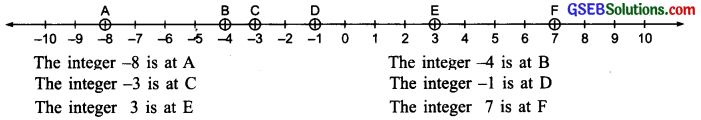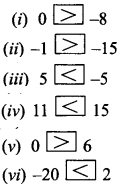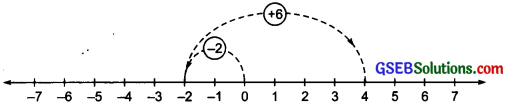# GSEB Solutions Class 6 Maths Chapter 6 Integers InText Questions

Gujarat Board GSEB Textbook Solutions Class 6 Maths Chapter 6 Integers InText Questions and Answers.

## Gujarat Board Textbook Solutions Class 6 Maths Chapter 6 Integers InText Questions

Try These (NCERT Page 116)

Question 1.
Write the following numbers with appropriate signs:
(a) 100 m below sea level.
(b) 25°C above 0°C temperature.
(c) 15°C below 0°C temperature.
(d) Any five numbers less than 0.
Solution:
(a) 100 m below sea level → – 100 m
(b) 25° above 0°C temperature → + 25° C
(c) 15°C below 0°C temperature → – 15° C
(d) Five numbers less than 0: { – 1, – 3, – 10, – 25, – 105 }
Note:
(i) If profit is represented by ‘+‘ sign, then loss may be represented by ‘-‘ sign.
(ii) If going up is represented by ‘+‘ sign, then going down may be represented by ‘-‘ sign.
(iii) If earnings are represented by ‘+‘ sign, then spending may be represented by ‘-‘ sign.
(iv) If temperature above 0° is ‘+‘ sign, then temperature below 00 may be represented by ‘-‘ sign.
(v) If depositing money to bank is ‘+‘ sign, then withdrawal is ‘-‘ sign.

Try These (NCERT Page 118)

Question 1.
Mark 3, 7, – 4, – 8, – 1 and – 3 on the number line.
Solution:
Given numbers are marked on the number line as shown below:Note: Look at the number line given here. We find that for every integer to the right of zero there is a corresponding integer to the left of zero (at the same distance from zero but with a negative sign). Similarly, for every integer to the left of zero. there is a corresponding integer to the right of zero (at the same distance from zero but with a positive sign).Try These (Page 119)

Question 1.
Compare the following pairs of numbers using> or <.Solution:Try These (Page 125)

Question 1.
Draw a figure on the ground in the form of a horizontal number line as shown below. Frame questions as given in the said example and ask your friends.Solution:
Do it yourself.

Try These (Page 125)

Question 1.
(a) ( -11) + ( -12)
(b) (+10) +(+4)
(c) (-32) + (-25)
(d) (+23) + (+40)
Solution:
(a) (-11) + (-12) = -[11 + 12] = -23
(b) (+10) + (+4) = + [10 + 4] +  = 14
(c) (- 32) + (- 25) = – [32 + 25] –  = -57
(d) (+23) + (40) = + [23 + 40] = +  = 63Try These (Page 125).

Question 1.
Find the solution of the following:
(a) (- 7) + (+8)
(b) (-9) + (+13)
(c) (+7) + (-10)
(d) (+12) + (-7)
Solution:
(a) (-7) + (+8):
Opposite of (-7) is (+7) and (+8)
= (+7) + (+1)
(- 7) + (+ 8) = (- 7) + (+7) + (+1)
= 0 + (+1) [(-7) + (+7) = 0]
= + 1

(b) (-9) + (+13):
(+13) – (+9) + (+4)
(-9) + (+ 13) = (-9) + (+9) + (+4)
= 0 + (+4) [(-9) + (+9) = 0]
= + 4 = 4

(c) (+7) + (-10):
(-10) = (-7) + (-3)
(+7) + (-10) = (+7) + (-7) + (-3)
= 0 + (-3)
[(+7) + (-7) = 0]
= – 3

(e) (+12) + (-7):
(+12) = (±7) + (+5)
(+12) + (-7) = (+7) + (+5) + (-7)
= (+7) + (-7) + (+5)
= – 0 + (+ 5)
= (+5)
= 5
[(+7) + (-7) = 0]Try These (Page 127)

Question 1.
Find the solution of thé following additions using a number line.
(a) (-2) + 6
(b) (-6) + 2
Make two such questions and solve them using the number line.
Solution:
(a) (-2) + 6First move 2 steps to the left of 0 to reach at – 2. From here, move 6 steps to the right of – 2, to reach at 4.
(-2) + (+6) = + 4

(b) (-6) + 2:On the number line, we first move 6 equal steps (each of 1 unit) to the left of 0, to reach at (-6). Now, move 2 steps to the right of (- 6) to reach at (- 4).
(-6) + (+2) = – 4Question 2.
Find the solution of the following without using number line:
(a) (+7) + (-11)
(b) (-13) + (+10)
(c) (-7) + (+9)
(d) (+10) + (-5)
Make five such questions and solve them.
(a) (+7) + (-11):
(-11) = (-7) + (-4)
(+7) + (-11) = (+7) + (-7) + (-4) = 0 + (-4)
[(+7) + (-7) = 0] = – 4
Thus, (+7) + (-11) = – 4

(b) (-13) + (+10):
(-13) = (-10) + (-3)
(-13) + (+10) = (-10) + (-3) + (+10)
= (-10) + (+10) + (-3)
= 0 + (-3) = -3
[(-10) + (+ 10) = 0]
Thus, (- 13) + (+10) = – 3

(c) (-7) + (+9):
(+9) = (+7) + (+2)
(-7) + (+9) = (-7) + (+7) + (+2) = 0 + (+2)
[(- 7) + (+7) = 0]
= + 2
Thus, (- 7) + (+9) = + 2

(d) (+10) + (-5):
(+10) = (+5) + (+5)
(+10) + (-5) = (+5) + (+5) + (-5) = + 5 + 0
[(+5) + (-5) = 0]
Thus, (+10) + (-5) = (+5)